StatLect

# R squared of a linear regression

How good is a linear regression model in predicting the output variable on the basis of the input variables?

How much of the variability in the output is explained by the variability in the inputs of a linear regression?

The R squared of a linear regression is a statistic that provides a quantitative answer to these questions.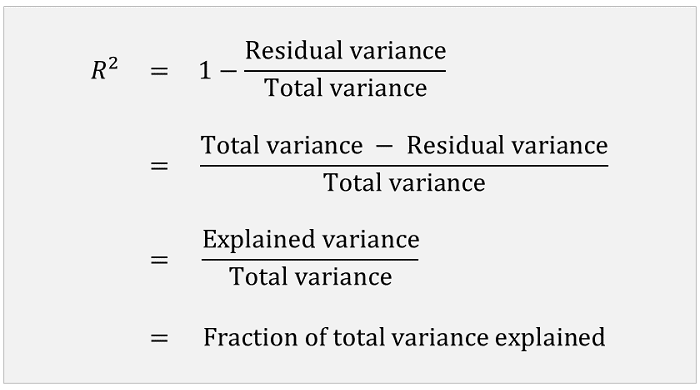## Caveat

Before defining the R squared of a linear regression, we warn our readers that several slightly different definitions can be found in the literature.

Usually, these definitions are equivalent in the special, but important case in which the linear regression includes a constant among its regressors.

We choose a definition that is easy to understand, and then we make some brief comments about other definitions.

## The linear regression model

Consider the linear regression modelwhereis avector of inputs andis avector of regression coefficients.

Suppose that we have a sample ofobservations, for.

Given an estimateof(for example, an OLS estimate), we compute the residuals of the regression:## Sample variance of the outputs

Denote bythe unadjusted sample variance of the outputs:whereis the sample meanThe sample varianceis a measure of the variability of the outputs, that is, of the variability that we are trying to explain with the regression model.

## Sample variance of the residuals

Denote bythe mean of the squared residuals: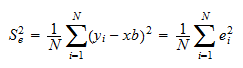which coincides with the unadjusted sample variance of the residuals when the sample mean of the residualsis equal to zero.

Unless stated otherwise, we are going to maintain the assumption thatin what follows.

The sample varianceis a measure of the variability of the residuals, that is, of the part of the variability of the outputs that we are not able to explain with the regression model.

Intuitively, when the predictions of the linear regression model are perfect, then the residuals are always equal to zero and their sample variance is also equal to zero.

On the contrary, the less the predictions of the linear regression model are accurate, the highest the variance of the residuals is.

## Definition of R squared

We are now ready to give a definition of R squared.

Definition The R squared of the linear regression, denoted by, iswhereis the sample variance of the residuals andis the sample variance of the outputs.

Thus, the R squared is a decreasing function of the sample variance of the residuals: the higher the sample variance of the residuals is, the smaller the R squared is.

## Properties and interpretation

Note that the R squared cannot be larger than 1: it is equal to 1 when the sample variance of the residuals is zero, and it is smaller than 1 when the sample variance of the residuals is strictly positive.

The R squared is equal to 0 when the variance of the residuals is equal to the variance of the outputs, that is, when predicting the outputs with the regression model is no better than using the sample mean of the outputs as a prediction.

It is possible to prove that the R squared cannot be smaller than 0 if the regression includes a constant among its regressors andis the OLS estimate of(in this case we also have that). Outside this important special case, the R squared can take negative values.

In summary, the R square is a measure of how well the linear regression fits the data (in more technical terms, it is a goodness-of-fit measure): when it is equal to 1 (and), it indicates that the fit of the regression is perfect; and the smaller it is, the worse the fit of the regression is.

## Alternative definition

Another common definition of the R squared is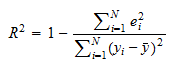This definition is equivalent to the previous definition in the case in which the sample mean of the residualsis equal to zero (e.g., if the regression includes an intercept).

Check the Wikipedia article for other definitions.

The adjusted R squared is obtained by using the adjusted sample variances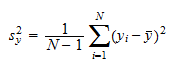and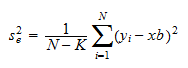instead of the unadjusted sample variancesand.

This is done becauseandare unbiased estimators ofandunder certain assumptions (see the lectures on Variance estimation and The Normal Linear Regression Model).

Definition The adjusted R squared of the linear regression, denoted by, iswhereis the adjusted sample variance of the residuals andis the adjusted sample variance of the outputs.

The adjusted R squared can also be written as a function of the unadjusted sample variances:Proof

This is an immediate consequence of the fact that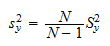and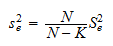The ratioused in the formula above is often called a degrees-of-freedom adjustment.

## Interpretation of the adjusted R squared

The intuition behind the adjustment is as follows.

When the numberof regressors is large, the mere fact of being able to adjust many regression coefficients allows us to significantly reduce the variance of the residuals. As a consequence, the R squared tends to be small.

This phenomenon is known as overfitting. The extreme case is when the number of regressorsis equal to the number of observationsand we can chooseso as to make all the residuals equal to.

But being able to mechanically make the variance of the residuals small by adjustingdoes not mean that the variance of the errors of the regressionis as small.

The degrees-of-freedom adjustment allows us to take this fact into consideration and to avoid under-estimating the variance of the error terms.

In more technical terms, the idea behind the adjustment is that what we would really like to know is the quantitybut the unadjusted sample variancesandare biased estimators ofand.

The bias is downwards, that is, they tend to underestimate their population counterparts.

As a consequence, we estimateandwith the adjusted sample variancesand, which are unbiased estimators.